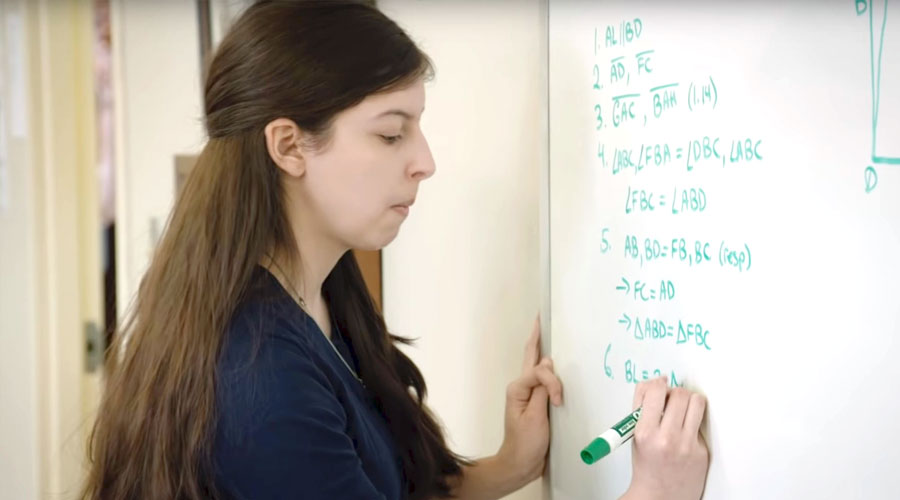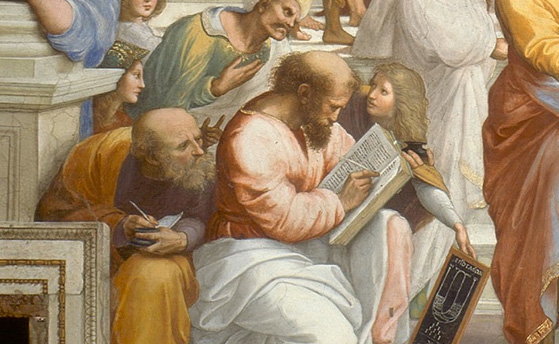(216) 707-1121
·The Lyceum's mathematics curriculum

### Pre-Algebra

For those students entering in middle school, we offer a Pre-Algebra course which teaches them all the topics they will need in order to excel at algebra. These topics include:
• fractions and their arithmetic operations
• decimals and their arithmetic operations
• mixed numbers and their arithmetic operations
• signed numbers and their arithmetic operations
• translating from words to algebraic expressions
• order of operations
• percents
• proportions
• ratios
• divisibility
• rounding
• place value
• unit conversions
• scientific notation
• data representation
• evaluation of algebraic expressions
• the simplification of algebraic expressions
• the solution of linear equations in one unknown
• word problems involving pre-algebraic concepts
• perimeter
• area
• surface area
• volume
• classification of geometric figures and solids
• geometric constructions
• symmetry

### Algebra 1

Algebra 1 continues these topics and introduces:
• arithmetic and evaluation of expressions involving signed numbers, exponents, and roots
• properties of real numbers
• absolute value
• equations and inequalities involving absolute value
• scientific notation
• unit conversions
• solution of equations in one unknown
• solution of simultaneous equations
• the algebra of polynomials and rational expressions
• word problems requiring algebra for the solution (such as uniform motion and coin problems)
• graphical solution of simultaneous equations
• graphs of a variety of functions: linear, quadratic, cubic, square root, absolute value, etc.
• translations and reflections of graphs
• factoring
• Pythagorean theorem
• algebraic proofs
• functional notation and functions
• solution of quadratic equations by factoring, completing the square, and quadratic formula
• direct and inverse variation
• exponential growth
• computation of the perimeter and area of two-dimensional regions
• computation of the surface area and volume of a wide variety of geometric solids
• statistics
• probability

### Geometry

Lyceum students learn Geometry from the second most widely published book of all time, surpassed only by the Holy Bible, Euclid's Elements. Since it was written, c. 300 BC, the Elements has been used by almost all great thinkers of western civilization to develop their ability to reason and use logic.

### Algebra 2

in addition to reviewing the topics learned in Algebra 1, introduces these concepts:
• graphical solution of simultaneous equations
• scientific notation
• roots of quadratic equations including complex roots
• properties of real numbers
• factoring
• inequalities and systems of inequalities
• logarithms and antilogarithms
• conic sections
• exponential equations
• basic trigonometric functions
• algebra of polynomials
• vectors in polar and rectangular form
• algebraic word problem

Advanced Mathematics continues the student's study of algebra and begins to introduce them to concepts which will aid them in the study of Calculus. The following material is introduced:
• permutations and combinations
• inverse trigonometric functions
• conic sections
• graphs of sinusoids
• rectangular and polar representation of complex numbers
• trigonometric identities
• De Moivre's theorem
• matrices and determinants
• the binomial theorem
• the rational roots theorem

### Calculus

We offer Calculus for those students who demonstrate an above-average ability in mathematics. It is an AP Course, and students may earn college credit by taking the AP Calculus Test.

### The Lyceum Curriculum